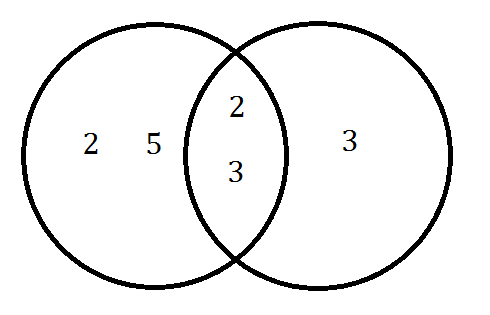Match Fishtank is now Fishtank Learning!

# Multi-Digit and Fraction Computation

## Objective

Find the least common multiple of two numbers. Solve application problems using the least common multiple.

## Common Core Standards

### Core Standards

?

• 6.NS.B.4 — Find the greatest common factor of two whole numbers less than or equal to 100 and the least common multiple of two whole numbers less than or equal to 12. Use the distributive property to express a sum of two whole numbers 1—100 with a common factor as a multiple of a sum of two whole numbers with no common factor. For example, express 36 + 8 as 4 (9 + 2).

?

• 4.OA.B.4

## Criteria for Success

?

1. Define and understand the least common multiple (LCM) as the smallest whole number that is a multiple of each number.
2. Find the least common multiple by listing and comparing the multiples of each number.
3. Find the least common multiple by finding the prime factorization of each number and identifying the nonrepeating factors of both numbers.
4. Determine the least common multiple of two relatively prime numbers is the product of the two numbers.
5. Solve word problems that involve finding the least common multiple.

## Tips for Teachers

?

#### Remote Learning Guidance

If you need to adapt or shorten this lesson for remote learning, we suggest prioritizing Anchor Problem 3 (benefits from worked example). Find more guidance on adapting our math curriculum for remote learning here.

#### Fishtank Plus

• Problem Set
• Student Handout Editor
• Vocabulary Package

## Anchor Problems

?

### Problem 1

1. List all the multiples of 8 that are less than or equal to 100.
2. List all the multiples of 12 that are less than or equal to 100.
3. What are the common multiples of 8 and 12 from the two lists?
4. What is the least common multiple of 8 and 12?
5. Lyle noticed that the list of common multiples has a pattern. Describe a pattern in the list of numbers that Lyle might have seen.

#### References

Illustrative Mathematics Multiples and Common Multiples

Multiples and Common Multiples, accessed on Sept. 28, 2017, 4:34 p.m., is licensed by Illustrative Mathematics under either the CC BY 4.0 or CC BY-NC-SA 4.0. For further information, contact Illustrative Mathematics.

### Problem 2

Find the least common multiple of 8 and 12 using prime factorization.

• Write 8 and 12 as products of prime factors using prime factorization.
• Draw a Venn diagram with prime factors of 8 in one circle and of 12 in another circle.
• Use the Venn diagram to find the least common multiple.

Use this same method to find the least common multiple of 12 and 9.

### Problem 3

Tre has two alarm clocks set to go off in the morning. One alarm will buzz every 6 minutes, and the other will beep every 9 minutes. Both alarms go off at 6:15 a.m.

Tre thinks the next time that both alarms will go off at the same time will be in 54 minutes or at 7:09. Do you agree with Tre? Explain your reasoning.

## Problem Set

?The following resources include problems and activities aligned to the objective of the lesson that can be used to create your own problem set.

• Include problems where students must determine two numbers that have a given least common multiple; for example: Name two numbers that have 20 as a least common multiple.
• Include examples of relatively prime numbers.
• Include examples where students find the GCF and LCM of the same pair of numbers.

?

### Problem 1

Find the least common multiple of 6 and 14. Show your work.

### Problem 2

A Venn diagram showing the prime factors for two numbers is shown below. Use it to answer the questions.1. What two numbers are represented in the diagram?
2. What is the greatest common factor of the two numbers?
3. What is the least common multiple of the two numbers?

?